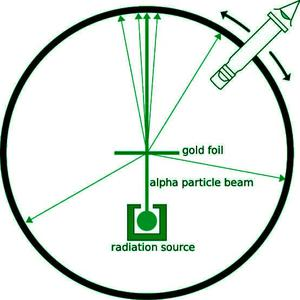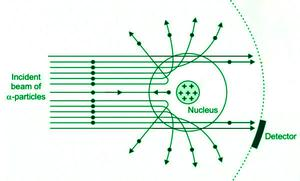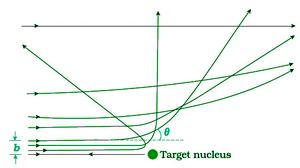Open in App
Not now

# Alpha Particle Scattering and Rutherford’s Nuclear Model of Atom

• Last Updated : 12 Oct, 2022

In today’s universe, the smallest particle of matter is an atom. It is the smallest possible result obtained by dividing the matter without releasing electrically charged particles. It was first proposed by John Dalton in the name of the Atomic Theory. This theory has its own evolution given by different scientists like J.J Thompson, Ernest Rutherford, Niels Bohr and Erwin Schrödinger. Today well will discuss the Rutherford Model of Atom.

### Rutherford Model

Ernest Rutherford was working on the emission of α-particles by the atoms to understand the structure of atoms and with respect to the experiment Rutherford was conducting Geiger and Marsden performed some experiments of their own as shown in the figure.Experimental setup of Geiger-Marsden experiment

They used a beam of 5.5 MeV emitted from a radioactive source (Bismuth) at a thin layer of foil made of gold. α-particles emitted by the source are concentrated into a single beam of light which was allowed to strike the thing gold foil and the concentrated beam is scattered after hitting and can be seen in the screen through a microscope.

This experiment was conducted and the result was that in the scattered beam of light, many particles pass through the foil without collusion, just 0.14% of incident particles scatter more than 1o and 1 in 8000 particles deflect more than 90o. This means that the particle was scattered completely to the backward direction which means there must be some kind of big repulsive forceWhy did that 1 in 8000 deflect?

Rutherford said from the observation that the greater repulsive force can be provided if the mass and positive charge is concentrated at the center which will cause the reflection.

So, in Rutherford’s model of the atom, all the positive charge and the mass are at the centre and the electrons will move around it like planets around the sun at some distance.

Rutherford said the size of the atom will be 10-15 approximately but in actual it was 10-10 and as electrons move around at some distance the atom is mostly empty space. Thus, because of such a large empty space in an atom, it is evident that most of the α-particles pass right through the atom and when any α-particles come near the nucleus, they are scattered due to the electric field.

### Scattering of the α-particles

As we all know, the gold foil is very thin, so we can assume that the α-particles will not suffer more than one collision. Thus the calculation was needed for a single nucleus. α-particles are nuclei of a helium atom, thus carrying 2 units (2e). The charge of the gold nucleus is Ze where Z is the atomic number of gold.

In this experiment, particles of gold are much heavier than an α-particle, the gold particles won’t move when the scattering occurs. The trajectory can be calculated by using Newton’s second law of motion and coulomb’s law of electrostatic force of repulsion between α-particles and positively charged nucleus which can be written as,

F = 1/(4πε0) × (2e)(Ze)/r2

where,

• r = distance between the α-particles and the nucleus.
• Ze = Charge of the gold nucleus

### Alpha-particle trajectoryThe trajectory of the alpha particle depends on the impact parameter ( the perpendicular distance of the initial velocity vector of the α-particle from the centre of the nucleus. in the above figure, there are various probabilities for the alpha particles to scatter on, and as you can see the α-particle close to the nucleus is the one that suffers the larger scattering. Now, the α-particle which hits the nucleus direct can face the scattering of even 180 degrees.

But through observation, it is evident that there are not many particles undergoing this 180-degree deviation because the number of particles returned was significant.

### Electron orbits in Rutherford’s Nuclear Model

The model involves classical concepts which are,

• It looks atom is an electrically neutral sphere.
• It has a very small, massive and positively charged nucleus at the centre.
• The nucleus at the centre is surrounded by revolving electrons.
• These electrons revolve in their respective orbits around the nucleus.
• The electrostatic force of attraction (Fe) between the revolving electrons and nucleus provides the required centripetal force (Fc) to maintain the rotation of the electrons around the nucleus]

Thus this can be represented as,

Fe – Fc

[1/(4πεo)] × [e2/r2] = mv2/r

thus the relation between the orbit radius and the electron velocity is

r = e2/(4πεomv2)

as we all know, kinetic energy (k) = 1/2 × (mv2)

then kinetic energy becomes,

K = e2/(8πεor)

Similarly, Potential energy (U) becomes,

U = -e2/(4πεo)

therefore total energy becomes the addition of kinetic and potential energy

T = K + U = [e2/(8πεor) + (-e2)/(4πεor))]

T = -e2/(8πεor)

thus, the total energy of electrons is negative which implies that electrons are electrically bonded to the nucleus.

### Sample Problems

Problem 1: In Rutherford’s nuclear model of the atom, the nucleus is analogous to the sun which is about which electrons move around the orbit (radius ≅ 10-10 m) like the earth orbits around the sun. If the dimensions of the solar system had the same proportions as those of the atom, would the earth be closer to or farther away from the sun than actually, it is? The radius of the earth’s orbit is about 1.5 × 1011 m. The radius of the sun is taken as 8 × 108

Solution:

The ratio of the radius of electron’s orbit to the radius of nucleus is (10-10m/10-15m) = 10

therefore it is seen that the radius of the electron’s orbit is 105 times larger than radius of nucleus.

If we consider the same situation for the earth and the sun i.e. earth’s orbit around sun were 105 times larger than radius of the sun, then the radius of the earth according this condition will be

105 × 8 × 108m = 8 × 1013 m which is without doubt prolonged than the actual orbit of earth and hence earth would be much farther away from the sun.

It also implies that an atom contains a much greater fraction of empty space as compared to our solar system.

Problem 2: It is experimentally found that 13.6 eV energy is required to separate a hydrogen atom into a proton and electron. Compute the orbital radius and the velocity of the electron in a hydrogen atom.

Solution:

Total energy of electron in hydrogen atom is -13.6 eV = -13.6 × 1.6 × 10-19  J

According to relation between orbit radius and electron velocity we get,

E = -e2/(8πεo r)

E = -2.2 × 10-18 J

r = -e2/(8πεo E)

r = [(9 ×109  N m2 /C2) (1.6 × 10-19 C)2 ] / [(2) (-2.2 × 10-18 J)]

r = 5.3 × 10-11 m.

Thus velocity of the electron can be computed by

v = e / (√4 πεomr)

v =2.2 × 106 m/s

### Conceptual Questions

Question 1: Which radioactive source was used in the Alpha-particle scattering experiment by Geiger and Marsden?

The radioactive element used in the alpha scattering experiment is an radioactive isotope of Bismuth (214 Bi 83).

Question 2: What was the ratio of the deflection in the Alpha-scattering experiment for 90o?

1 out of 8000 of the Alpha particles were deflected over 90o .

Question 3: Explain the behaviour of the Alpha particles when they hit the gold foil in the Alpha scattering experiment.

When the alpha particles from the source hit the gold foil, many of them pass straight through the gold foil, just about 0.14 % of the particles which collide with the nucleus scatter more than 1o, 1 in 8000 particle deflect more than 90° and Very few of them hit the nucleus head on and returned back to the source’s direction i.e. 180o.

Question 4: Which two laws are used to calculate the trajectory of the collided alpha particle?

The Newton’s second law of motion and coulomb’s law of electrostatic force of repulsion between α-particles and positively charged nucleus.

Question 5: State any two properties of an atom given by Rutherford’s Nuclear Model.

The properties of an atom given by Rutherford’s Nuclear Model are:

1. The nucleus at the center is surrounded by revolving electrons.
2. These electrons revolves at their respective orbits around nucleus.

Question 6: Why did the Alpha particles change the trajectory when collided with the nucleus? Why didn’t the Alpha particles?